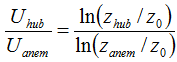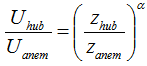# HOMER Grid 1.4

 Navigation: Design > Resources > Wind Resource Wind Resource Variation with HeightUnder the Variation with Height tab on the Wind Resource page, you can describe the way the wind speed increases with height above ground. HOMER uses this information to calculate the wind speed at the hub height of the wind turbine.

Ground-level obstacles such as vegetation, buildings, and topographic features tend to slow the wind near the surface. Because the effect of these obstacles decreases with height above ground, wind speeds tend to increase with height above ground. This variation of wind speed with height is called wind shear. Wind energy engineers typically model wind shear using one of two mathematical models, the logarithmic profile or the power law profile.

Under Wind speed profile, select either the Logarithmic radio button or the Power law radio button.

## Logarithmic Profile

The logarithmic profile (or log law) assumes that the wind speed is proportional to the logarithm of the height above ground. The following equation therefore gives the ratio of the wind speed at hub height to the wind speed at anemometer height:where: Uhub = the wind speed at the hub height of the wind turbine [m/s] Uanem = the wind speed at anemometer height [m/s] zhub = the hub height of the wind turbine [m] zanem = the anemometer height [m] z0 = the surface roughness length [m] ln(..) = the natural logarithm

The surface roughness length is a parameter that characterizes the roughness of the surrounding terrain. The table below contains representative surface roughness lengths taken from Manwell, McGowan, and Rogers. Enter a value in the Surface roughness length (m) box.

 Terrain Description z0 Very smooth, ice or mud 0.00001 m Calm open sea 0.0002 m Blown sea 0.0005 m Snow surface 0.003 m Lawn grass 0.008 m Rough pasture 0.010 m Fallow field 0.03 m Crops 0.05 m Few trees 0.10 m Many trees, few buildings 0.25 m Forest and woodlands 0.5 m Suburbs 1.5 m City center, tall buildings 3.0 m

## Power Law Profile

The power law profile assumes that the ratio of wind speeds at different heights is given by the following equation:where: Uhub = the wind speed at the hub height of the wind turbine [m/s] Uanem = the wind speed at anemometer height [m/s] zhub = the hub height of the wind turbine [m] zanem = the anemometer height [m] α = the power law exponent

The power law exponent is a dimensionless parameter. Foundational research in fluid mechanics showed that its value is equal to 1/7 for turbulent flow over a flat plate. Wind speed researchers have found that, in practice, the power law exponent depends on terrain roughness, atmospheric stability, and several other factors. Enter a value in the Power law exponent box.